# JP2017203683A - Capacitance type triaxial acceleration sensor - Google Patents

## Info

Publication number
JP2017203683A
JP2017203683A JP2016095242A JP2016095242A JP2017203683A JP 2017203683 A JP2017203683 A JP 2017203683A JP 2016095242 A JP2016095242 A JP 2016095242A JP 2016095242 A JP2016095242 A JP 2016095242A JP 2017203683 A JP2017203683 A JP 2017203683A
Authority
JP
Japan
Prior art keywords
direction
electrode
divided
acceleration
divided electrode
Prior art date
Legal status (The legal status is an assumption and is not a legal conclusion. Google has not performed a legal analysis and makes no representation as to the accuracy of the status listed.)
Pending
Application number
JP2016095242A
Other languages
Japanese (ja)
Inventor

Eiji Tamakoshi

Takashi Kawasaki

Tan Nakamura

Original Assignee

Naigai Rubber Kk
Priority date (The priority date is an assumption and is not a legal conclusion. Google has not performed a legal analysis and makes no representation as to the accuracy of the date listed.)
Filing date
Publication date
Application filed by 内外ゴム株式会社, Naigai Rubber Kk filed Critical 内外ゴム株式会社
Priority to JP2016095242A priority Critical patent/JP2017203683A/en
Publication of JP2017203683A publication Critical patent/JP2017203683A/en
Application status is Pending legal-status Critical

• 230000001133 acceleration Effects 0 abstract title 4
• 238000006073 displacement Methods 0 abstract 3
• 230000001965 increased Effects 0 abstract 1
• 230000035945 sensitivity Effects 0 abstract 1

## Images

•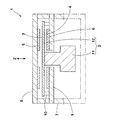•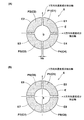•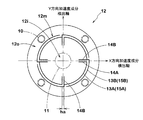••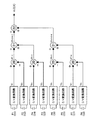••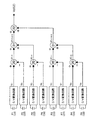•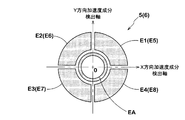•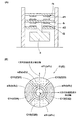## Abstract

PROBLEM TO BE SOLVED: To heighten sensor sensitivity by increasing an electrode area, while maintaining a sensor size.SOLUTION: A triaxial acceleration sensor includes a weight flexible member 2 having a flexible plate 12, first and second displacement electrodes 3, 4 arranged on each surface on one side/the other side of the flexible plate 12, a first fixed electrode 5 facing to the first displacement electrode 3, and a second fixed electrode 6 facing to the second displacement electrode 4. The first and second fixed electrodes 5, 6 include four segment-shaped split electrode pieces E split respectively by an X-direction acceleration component detection axis and a Y-direction acceleration component detection axis.SELECTED DRAWING: Figure 1

## Description

The present invention relates to a capacitance type triaxial acceleration sensor capable of improving detection accuracy.

As a capacitance type triaxial acceleration sensor, for example, one described in Patent Document 1 below is known. As shown schematically in FIG.
(1) a flexible plate b to which a weight a is attached;
(2) first and second displacement electrodes d1, d2 disposed on one surface and the other surface of the flexible plate b;
(3) First and second fixed electrodes f1 and f2 facing the first and second displacement electrodes d1 and d2, respectively.

As shown in FIG. 9 (B), the first fixed electrode f1 is a pair of electrode pieces eX1, eX2, Y direction dedicated for X-direction acceleration measurement placed on both sides of the origin 0 on the X-direction acceleration component detection axis. It is formed from a pair of electrode pieces eY1 and eY2 dedicated to Y-direction acceleration measurement that are placed on the acceleration component detection axis and on both sides of the origin 0, and an electrode piece eZ1 dedicated to acceleration measurement in the Z direction arranged at the position of the origin 0. The Similarly, the second fixed electrode f2 also has a pair of electrode pieces eX3 and eX4 dedicated for X-direction acceleration measurement placed on the X-direction acceleration component detection axis and on both sides of the origin 0, on the Y-direction acceleration component detection axis and the origin. A pair of electrode pieces eY3 and eY4 dedicated to the acceleration measurement in the Y-direction acceleration component detection axis direction that face each other on the zero side, and an electrode piece eZ2 dedicated to the Z-direction acceleration measurement arranged at the position of the origin 0.

The capacitance value of the electrode piece eZ1 is c11, the capacitance values of the electrode pieces eX1 and eX2 are c12 and c14, the capacitance values of the electrode pieces eY1 and eY2 are c13 and c15, and the capacitance value of the electrode piece eZ2. When the value is c21, the capacitance values of the electrode pieces eX3 and eX4 are c22 and c24, and the capacitance values of the electrode pieces eY3 and eY4 are c23 and c25, static corresponding to the accelerations GX, GY, and GZ in the XYZ directions. The capacitance values cX, cY, cZ are calculated by the following equations (1 ′) to (3 ′), and the respective accelerations GX, GY, GZ are detected.
cX = (c12-c14)-(c22-c24)-(1 ')
cY = (c13-c15)-(c23-c25)-(2 ')
cZ = (c11)-(c21)-(3 ')

In this three-axis acceleration sensor, when accelerations in the X direction, Y direction, and Z direction act independently, the sensitivity is about 2 as compared with the case where there is no second displacement electrode d2 and second fixed electrode f2. Can be doubled. Even when acceleration in the Z direction acts simultaneously with the X direction (or Y direction), the influence of the acceleration in the Z direction can be eliminated, and the detection accuracy can be improved.

However, in view of the recent demand for higher performance, further improvement in detection accuracy is strongly desired. The capacitance value C is given by the following equation from the dielectric constant ε, the electrode area S, and the interelectrode distance d.
C = ε × S / d
Therefore, by increasing the electrode area S, it is possible to increase the sensor sensitivity that is the capacitance value C. However, on the other hand, there is a problem of increasing the sensor size, and there is a limit to the increase in the electrode area S.

Japanese Patent Laid-Open No. 11-133305

Therefore, the present invention can increase the electrode area while maintaining the sensor size, based on the fact that each electrode piece is used for the acceleration measurement in the X direction, the acceleration measurement in the Y direction, and the acceleration measurement in the Z direction. An object of the present invention is to provide a capacitance type three-axis acceleration sensor capable of improving the detection accuracy by increasing the sensor sensitivity.

The present invention is a three-axis acceleration sensor that detects acceleration in the XYZ directions,
A weight flexible member having a flexible plate to which a weight is attached and which is displaced by acceleration acting on the weight,
A first displacement electrode disposed on one surface of the flexible plate in the Z direction, and a second displacement electrode disposed on the other surface;
And a first fixed electrode facing the first displacement electrode, and a second fixed electrode facing the second displacement electrode and arranged in parallel with each other, and
Each of the first and second fixed electrodes includes four segment-shaped divided electrode pieces that are divided by an X-direction acceleration component detection axis and a Y-direction acceleration component detection axis and are electrically independent.

In the capacitance type triaxial acceleration sensor according to the present invention, it is preferable that the first and second fixed electrodes are each composed of four divided electrode pieces.

In the capacitance type triaxial acceleration sensor according to the present invention, it is preferable that the divided electrode pieces have the same shape.

In the capacitance type triaxial acceleration sensor according to the present invention, the divided electrode piece of the first fixed electrode is a first divided electrode having a capacitance value C1 located in the first quadrant in the XY coordinate system. The piece E1, the second divided electrode piece E2 having a capacitance value C2 located in the second quadrant, the third divided electrode piece E3 having the capacitance value C3 located in the third quadrant, and the fourth quadrant A fourth divided electrode piece E4 having a capacitance value C4;
The divided electrode pieces of the second fixed electrode are divided into a fifth divided electrode piece E5 having a capacitance value C5 located in the first quadrant and a capacitance value located in the second quadrant in the XY coordinate system. From the sixth divided electrode piece E6 of C6, the seventh divided electrode piece E7 of the capacitance value C7 located in the third quadrant, and the eighth divided electrode piece E8 of the capacitance value C8 located in the fourth quadrant As
Any one of the capacitance value CX corresponding to the acceleration GX in the X direction, the capacitance value CY corresponding to the acceleration GY in the Y direction, and the capacitance value CZ corresponding to the acceleration GZ in the Z direction It is preferable to calculate based on the capacitance values C1, C2, C3, C4, C5, C6, C7, and C8 and detect any one of accelerations GX, GY, and GZ acting on the weight.

In the capacitance type triaxial acceleration sensor according to the present invention, any one of the capacitance values CX, CY, and CZ is preferably calculated based on the following equations (1) to (3).
CX = (C1 + C4)-(C2 + C3) + (C6 + C7)-(C5 + C8) --- (1)
CY = (C1 + C2)-(C3 + C4) + (C7 + C8)-(C5 + C6) --- (2)
CZ = (C1 + C4) + (C2 + C3)-(C5 + C8)-(C6 + C7) --- (3)

In the capacitance type triaxial acceleration sensor according to the present invention, the flexible plate includes an origin position where the X direction acceleration component detection axis and the Y direction acceleration component detection axis intersect, and a central region to which the weight is attached. An outer peripheral region to which the flexible plate is fixed, and a flexible region between the central region and the outer peripheral region,
The flexible region has radially inner and outer arc-shaped slits with the origin as the arc center, and the radially inner and outer arc-shaped slits are divided slits each divided into four equal parts in the circumferential direction,
The split slit forming the arcuate slit on the radially inner side is provided with a first radial slit portion extending radially inward from one circumferential end thereof,
The split slit forming the radially outer arc-shaped slit is provided with a second radial slit portion extending radially inward from one circumferential end thereof,
Moreover, the first and second radial slit portions face each other on both sides of the X-direction acceleration component detection axis or the Y-direction acceleration component detection axis,
It is preferable that the other end in the circumferential direction of the split slit forming the arcuate slit on the radially inner side is connected to the second radial slit.

In the present invention, as described above, the first and second fixed electrodes are formed to include four segment-shaped divided electrode pieces each divided by an X-direction acceleration component detection axis and a Y-direction acceleration component detection axis. The

In this case, in each of the first and second fixed electrodes, out of four divided electrode pieces, two divided electrode pieces arranged on one side of the Y-direction acceleration component detection axis and two arranged on the other side The divided electrode piece can be used as an electrode for acceleration measurement in the X direction.

In the first and second fixed electrodes, of the four divided electrode pieces, two divided electrode pieces arranged on one side of the X-direction acceleration component detection axis and two divided electrodes arranged on the other side The piece can be used as an electrode for acceleration measurement in the Y direction.

Also, the four divided electrode pieces in the first fixed electrode and the four divided electrode pieces in the second fixed electrode can be used as electrodes for acceleration measurement in the Z direction.

That is, the first and second fixed electrodes are divided into four parts by the X-direction acceleration component detection axis and the Y-direction acceleration component detection axis, respectively, so that each divided electrode piece is measured in the X direction and Y direction. , And Z-direction acceleration measurement.

Accordingly, as shown in the conventional example of FIG. 9B, when the four electrode pieces are divided into two electrode pieces dedicated to acceleration measurement in the X direction and two electrode pieces dedicated to acceleration measurement in the Y direction. In comparison, the electrode area S can be increased approximately twice, and the sensor sensitivity can be increased while maintaining the sensor size, and the detection accuracy can be improved.

It is sectional drawing which shows one Embodiment of the 3-axis acceleration sensor of this invention. (A), (B) is a top view which shows a fixed electrode. It is a top view which shows a flexible plate. (A)-(C) are drawings which show the combination of the division | segmentation electrode piece for detecting the acceleration of an XY direction. It is a circuit diagram of the calculating part of a X direction. It is a circuit diagram of the calculating part of a Y direction. It is a circuit diagram of the calculating part of a Z direction. It is a top view which shows the other example of the 1st, 2nd fixed electrode. (A) Sectional drawing which shows the conventional triaxial acceleration sensor, (B) is a top view which shows a fixed electrode.

Hereinafter, embodiments of the present invention will be described in detail.
As shown in FIG. 1, the capacitance type triaxial acceleration sensor 1 of this embodiment includes a weight flexible member 2 and first and second displacement electrodes 3, 4 attached to the weight flexible member 2. And the first and second fixed electrodes 5 and 6 facing the first and second displacement electrodes 3 and 4, the acceleration in the Z direction which is the vertical direction, the X direction and the Y direction which are the horizontal directions Detect acceleration.

Specifically, the triaxial acceleration sensor 1 of this example includes a sensor housing 7 and upper and lower substrates 8 and 9 that are fixed to the sensor housing 7 and are parallel to each other. In this example, the weight flexible member 2 is supported on the lower substrate 9 via the boss portion 10. The weight flexible member 2 can be supported on the sensor casing 7 or the substrate 8 on the upper side.

The weight flexible member 2 includes a weight 11 and a disk-shaped flexible plate 12 to which the weight 11 is attached. The flexible plate 12 is disposed between the upper and lower substrates 8 and 9.

As shown in FIG. 3, the flexible plate 12 includes a central region 12i including the position of the origin O where the X-direction acceleration component detection axis and the Y-direction acceleration component detection axis intersect, and an outer peripheral region where the flexible plate 12 is fixed. 12o and a flexible region 12m between the central region 12i and the outer peripheral region 12o. The weight 11 is attached to the central region 12i. In addition, the outer peripheral region 12o is fixed to the substrate 9 through the boss portion 10 in this example.

The flexible region 12m includes arc-shaped slits 13A and 13B in the radial direction with the origin O as the center of the arc, and first and second radial slit portions 14A and 14B. The inner arc-shaped slit 13A is composed of divided slits 15A divided into four equal parts in the circumferential direction, and the outer arc-shaped slit 13B is composed of divided slits 15B divided into four equal parts in the circumferential direction.

Each of the divided slits 15A is continuously provided with a first radial slit portion 14A extending radially inward from one circumferential end portion thereof. Each of the divided slits 15B is continuously provided with a second radial slit portion 14B extending radially inward from one circumferential end thereof. The first and second radial slit portions 14A and 14B face each other on both sides of the X-direction acceleration component detection axis or the Y-direction acceleration component detection axis.

The split slit 15A forming the inner arc-shaped slit 13A has the other circumferential end connected to the second radial slit 14B. By providing the arc-shaped slits 13A and 13B and the first and second radial slit portions 14A and 14B, the flexible region 12m is displaced in an arbitrary direction by the acceleration acting on the weight 11. sell.

The first displacement electrode 3 is disposed on the surface of one side (upper side in this example) of the flexible plate 12, and the second displacement electrode 4 is disposed on the other side (lower side in this example). Is arranged. In this example, the flexible plate 12 is formed of a conductive metal plate, and therefore the flexible plate 12 itself constitutes the first and second displacement electrodes 3 and 4.

In this example, the first fixed electrode 5 facing the first displacement electrode 3 is provided on the lower surface of the upper substrate 8, and the second fixed electrode facing the second displacement electrode 4 is disposed on the upper surface of the lower substrate 9. An electrode 6 is provided. The first and second fixed electrodes 5 and 6 are parallel to each other. In a reference state where gravity does not act, the distance between the displacement electrode 3 and the fixed electrode 5 and the distance between the displacement electrode 4 and the fixed electrode 6 are as follows. Are equal to each other.

As shown in FIGS. 2A and 2B, each of the first and second fixed electrodes 5 and 6 has four segment shapes divided by an X-direction acceleration component detection axis and a Y-direction acceleration component detection axis, respectively. It is formed including the divided electrode piece E. Each divided electrode piece E is electrically independent. In this example, a case where the first and second fixed electrodes 5 and 6 are each formed of four divided electrode pieces E is shown. It is preferable that the divided electrode pieces E have the same shape.

Specifically, the divided electrode piece E of the first fixed electrode 5 includes a first divided electrode piece E1 located in the first quadrant and a second divided electrode piece located in the second quadrant in the XY coordinate system. E2, a third divided electrode piece E3 located in the third quadrant, and a fourth divided electrode piece E4 located in the fourth quadrant.

The divided electrode piece E of the second fixed electrode 6 includes, in the XY coordinate system, a fifth divided electrode piece E5 located in the first quadrant, a sixth divided electrode piece E6 located in the second quadrant, It consists of a seventh divided electrode piece E7 located in the third quadrant and an eighth divided electrode piece E8 located in the fourth quadrant.

As a result, the first displacement electrode 3 and the first divided electrode piece E1 form a capacitance element P1 having a capacitance value C1, and the first displacement electrode 3 and the second divided electrode piece E2 form an electrostatic capacitance. A capacitance element P2 having a capacitance value C2 is formed, and a capacitance element P3 having a capacitance value C3 is formed by the first displacement electrode 3 and the third divided electrode piece E3. A capacitive element P4 having a capacitance value C4 is formed by the fourth divided electrode piece E1.

Similarly, a capacitance element P5 having a capacitance value C5 is formed by the second displacement electrode 4 and the fifth divided electrode piece E5, and electrostatic capacitance is formed by the second displacement electrode 4 and the sixth divided electrode piece E6. A capacitance element P6 having a capacitance value C6 is formed, and a capacitance element P7 having a capacitance value C7 is formed by the second displacement electrode 4 and the seventh divided electrode piece E7. A capacitive element P8 having a capacitance value C8 is formed by the eighth divided electrode piece E8.

Each of the divided electrode pieces E1 to E8 has the same shape in this example and is made of the same metal material. In addition, the distance ha (shown in FIG. 3) between the first and second radial slit portions 14A and 14B adjacent to each other with the X-direction acceleration component detection axis or the Y-direction acceleration component detection axis interposed therebetween is the X-direction acceleration component detection. It is preferable that the distance is smaller than the interval hb between the circumferential ends of the adjacent divided electrode pieces E across the axis or the Y-direction acceleration component detection axis.

In such a triaxial acceleration sensor 1, the divided electrode pieces E can be combined as follows and used as an acceleration measuring electrode in the X direction. That is, in the fixed electrode 5, as shown in FIG. 4A, of the four divided electrode pieces E1 to E4, two divided electrode pieces E1 and E4 arranged on one side of the Y-direction acceleration component detection axis. The combination R14 and the combination R23 of the two divided electrode pieces E2 and E3 arranged on the other side can be used as an acceleration measuring electrode in the X direction. Similarly, in the fixed electrode 6, as shown in FIG. 4B, among the four divided electrode pieces E5 to E8, two divided electrode pieces E5 arranged on one side of the Y-direction acceleration component detection axis, The set R58 of E8 and the set R67 of two divided electrode pieces E6 and E7 arranged on the other side can be used as electrodes for measuring the acceleration in the X direction.

When used as an acceleration measuring electrode in the Y direction, the fixed electrode 5 is arranged on one side of the X direction acceleration component detection axis among the four divided electrode pieces E1 to E4 as shown in FIG. The set R12 of the two divided electrode pieces E1 and E2 and the set R34 of the two divided electrode pieces E3 and E4 arranged on the other side can be used as electrodes for acceleration measurement in the Y direction. Similarly, in the fixed electrode 6, as shown in FIG. 4D, of the four divided electrode pieces E5 to E8, two divided electrode pieces E5 arranged on one side of the X-direction acceleration component detection axis, The set R56 of E6 and the set R78 of two divided electrode pieces E7 and E8 arranged on the other side can be used as electrodes for measuring the acceleration in the Y direction.

Although not shown, as the electrodes for measuring the acceleration in the Z direction, the combinations R1 to 4 of the four divided electrode pieces E1 to E4 and the sets R5 to 8 of the four divided electrode pieces E5 to E8 can be used.

Therefore, in contrast to the above-described three-axis acceleration sensor of Patent Document 1 shown in FIGS. 9A and 9B, each of the divided electrode pieces E can be used as follows in the three-axis acceleration sensor 1 of the present invention.
Electrode piece eX1 → Divided electrode piece (E1 + E4)
Electrode piece eX2 → Divided electrode piece (E2 + E3)
Electrode piece eX3 → Divided electrode piece (E5 + E8)
Electrode piece eX4 → Divided electrode piece (E6 + E7)
Electrode piece eY1 → Divided electrode piece (E1 + E2)
Electrode piece eY2 → Divided electrode piece (E3 + E4)
Electrode piece eY3 → Divided electrode piece (E5 + E6)
Electrode piece eY4 → Divided electrode piece (E7 + E8)
Electrode piece eZ1 → Divided electrode piece (E1 + E2 + E3 + E4)
Electrode piece eZ2 → Divided electrode piece (E5 + E6 + E7 + E8)

That is, in the present invention, the electrode area can be increased about twice as much as the case of Patent Document 1 as the acceleration measuring electrode in the X direction and the acceleration measuring electrode in the Y direction. While maintaining the sensor sensitivity, the detection accuracy can be improved by increasing the sensor sensitivity. In addition, the electrode area can be greatly increased for the acceleration measuring electrode in the Z direction as compared with the case of Patent Document 1.

As shown in FIG. 8, as the first and second fixed electrodes 5 and 6, electrodes EA dedicated to acceleration measurement in the Z direction can be provided inside the four divided electrode pieces E in the radial direction. However, when the electrode EA is not provided and the first and second fixed electrodes 5 and 6 are configured by only the four divided electrode pieces E, the electrode area of the divided electrode piece E can be increased by the amount of the electrode EA. Therefore, the sensor sensitivity can be further improved.

The triaxial acceleration sensor 1 includes a calculation unit (not shown) that detects accelerations GX, GY, and GZ acting on the weight 11 from the capacitance values C1 to C8 of the capacitance elements P1 to P8. Yeah.

The arithmetic unit is, for example, an electric circuit formed on the substrates 8 and 9, and any one of the capacitance values CX, CY, and CZ corresponding to the accelerations GX, GY, and GZ is set to all the capacitances. Calculation is made based on the values C1, C2, C3, C4, C5, C6, C7, and C8, and any one of the accelerations GX, GY, and GZ is detected. In this example, the capacitance values CX, CY, and CZ are calculated based on all the capacitance values C1, C2, C3, C4, C5, C6, C7, and C8, and the accelerations GX and GY are calculated. , GZ is detected. The calculation method is not particularly specified.

However, when each of the divided electrode pieces E1 to E8 is made of the same shape and the same metal material as in this example, it can be calculated based on the following equations (1) to (3).
CX = (C1 + C4)-(C2 + C3) + (C6 + C7)-(C5 + C8) --- (1)
CY = (C1 + C2)-(C3 + C4) + (C7 + C8)-(C5 + C6) --- (2)
CZ = (C1 + C4) + (C2 + C3)-(C5 + C8)-(C6 + C7) --- (3)

The above formulas (1) to (3) correspond to the following formulas (1 ′) to (3 ′) of Patent Document 1 described above.
cX = (c12-c14)-(c22-c24) --- (1 ')
cY = (c13-c15)-(c23-c25) --- (2 ')
cZ = (c11)-(c21) --- (3 ')

Therefore, the triaxial acceleration sensor 1 can further increase the electrode area while exhibiting the advantages of Patent Document 1, and can increase the sensor sensitivity and improve the detection accuracy while maintaining the sensor size. it can. The advantages and mechanism of Patent Document 1 are as described in Patent Document 1, and the description thereof is omitted in this specification.

Next, an example of the circuit configuration of the arithmetic unit is shown in FIGS. 5 calculates the output of the acceleration GX in the X direction, FIG. 6 calculates the output of the acceleration GY in the Y direction, and FIG. 7 calculates the output of the acceleration GZ in the Z direction.

For example, in FIG. 5, the capacitance values C <b> 1 to C <b> 8 are converted into voltage values V <b> 1 to V <b> 8 by C / V conversion circuits, respectively, and output to the differential amplifiers 20. Each differential amplifier 20 includes a voltage V 1-2 that is a difference between the voltage values V 1 and V 2 , a voltage V 4-3 that is a difference between the voltage values V 4 and V 3, and a voltage value V 6 and V 5 . The difference voltage V 6-5 and the difference voltage V 7-8 between the voltage values V 7 and V 8 are output to the summing amplifier 21, respectively. Each summing amplifier 21, the voltage V 1-2 and the voltage V 1-2 + 4-3 of the sum of the voltage V 4-3, and the voltage V 6-5 and the voltage of the sum of the voltage V 7-8 V 6-5 + 7 -8 it is output to the summing amplifier 22, whereby the voltage value V X corresponding to the acceleration GX equivalent to X direction to the electrostatic capacitance value CX is obtained.

The acceleration output CY in the Y direction and the acceleration output CZ in the Z direction are also obtained by using a C / V conversion circuit, a differential amplifier 20, and an addition amplifier 21, as shown in FIGS. Desired.

As mentioned above, although especially preferable embodiment of this invention was explained in full detail, this invention is not limited to embodiment of illustration, It can deform | transform and implement in a various aspect.

1 axis acceleration sensor 2 weight flexible member 3 first displacement electrode 4 second displacement electrode 5 first fixed electrode 6 second fixed electrode 11 weight 12 flexible plate 12i central side region 12m flexible region 12o Outer peripheral regions 13A and 13B Arc-shaped slits 14A in the radial direction and the outer radial regions 14A First radial slit portions 14B Second radial slit portions 15A and 15B Split slits

## Claims (6)

1. A triaxial acceleration sensor for detecting acceleration in the XYZ directions,
A weight flexible member having a flexible plate to which a weight is attached and which is displaced by acceleration acting on the weight,
A first displacement electrode disposed on one surface of the flexible plate in the Z direction, and a second displacement electrode disposed on the other surface;
And a first fixed electrode facing the first displacement electrode, and a second fixed electrode facing the second displacement electrode and arranged in parallel with each other, and
The first and second fixed electrodes each include four segment-shaped divided electrode pieces that are divided by an X-direction acceleration component detection axis and a Y-direction acceleration component detection axis and are electrically independent. Capacitance type 3-axis acceleration sensor.
2.   2. The capacitance type triaxial acceleration sensor according to claim 1, wherein each of the first and second fixed electrodes includes four divided electrode pieces.
3.   3. The capacitance type triaxial acceleration sensor according to claim 1, wherein the divided electrode pieces have the same shape.
4. The divided electrode piece of the first fixed electrode includes a first divided electrode piece E1 having a capacitance value C1 located in the first quadrant and a capacitance value C2 located in the second quadrant in the XY coordinate system. Second divided electrode piece E2, a third divided electrode piece E3 having a capacitance value C3 located in the third quadrant, and a fourth divided electrode piece E4 having a capacitance value C4 located in the fourth quadrant. ,
The divided electrode pieces of the second fixed electrode are divided into a fifth divided electrode piece E5 having a capacitance value C5 located in the first quadrant and a capacitance value located in the second quadrant in the XY coordinate system. From the sixth divided electrode piece E6 of C6, the seventh divided electrode piece E7 of the capacitance value C7 located in the third quadrant, and the eighth divided electrode piece E8 of the capacitance value C8 located in the fourth quadrant As
Any one of the capacitance value CX corresponding to the acceleration GX in the X direction, the capacitance value CY corresponding to the acceleration GY in the Y direction, and the capacitance value CZ corresponding to the acceleration GZ in the Z direction Calculated based on capacitance values C1, C2, C3, C4, C5, C6, C7, and C8, and detects any one of accelerations GX, GY, and GZ acting on the weight. The capacitance type triaxial acceleration sensor according to claim 3.
5. 5. The capacitance type triaxial acceleration sensor according to claim 4, wherein any one of the capacitance values CX, CY, and CZ is calculated based on the following equations (1) to (3): .
CX = (C1 + C4)-(C2 + C3) + (C6 + C7)-(C5 + C8) --- (1)
CY = (C1 + C2)-(C3 + C4) + (C7 + C8)-(C5 + C6) --- (2)
CZ = (C1 + C4) + (C2 + C3)-(C5 + C8)-(C6 + C7) --- (3)
6. The flexible plate includes an origin position where an X-direction acceleration component detection axis and a Y-direction acceleration component detection axis intersect, and a central region to which the weight is attached, an outer peripheral region to which the flexible plate is fixed, and the center Comprising a flexible region between the side region and the outer peripheral region,
The flexible region has radially inner and outer arc-shaped slits with the origin as the arc center, and the radially inner and outer arc-shaped slits are divided slits each divided into four equal parts in the circumferential direction,
The split slit forming the arcuate slit on the radially inner side is provided with a first radial slit portion extending radially inward from one circumferential end thereof,
The split slit forming the radially outer arc-shaped slit is provided with a second radial slit portion extending radially inward from one circumferential end thereof,
Moreover, the first and second radial slit portions face each other on both sides of the X-direction acceleration component detection axis or the Y-direction acceleration component detection axis,
The static slit according to any one of claims 1 to 5, wherein the other end in the circumferential direction of the split slit forming the arc-shaped slit on the radially inner side is connected to the second radial slit. Capacitance type 3-axis acceleration sensor.
JP2016095242A 2016-05-11 2016-05-11 Capacitance type triaxial acceleration sensor Pending JP2017203683A (en)

## Priority Applications (1)

Application Number Priority Date Filing Date Title
JP2016095242A JP2017203683A (en) 2016-05-11 2016-05-11 Capacitance type triaxial acceleration sensor

## Applications Claiming Priority (1)

Application Number Priority Date Filing Date Title
JP2016095242A JP2017203683A (en) 2016-05-11 2016-05-11 Capacitance type triaxial acceleration sensor

## Publications (1)

Publication Number Publication Date
JP2017203683A true JP2017203683A (en) 2017-11-16

# Family

## Family Applications (1)

Application Number Title Priority Date Filing Date
JP2016095242A Pending JP2017203683A (en) 2016-05-11 2016-05-11 Capacitance type triaxial acceleration sensor

## Country Status (1)

JP (1) JP2017203683A (en)

## Citations (10)

* Cited by examiner, † Cited by third party
Publication number Priority date Publication date Assignee Title
JPH05118942A (en) * 1991-10-25 1993-05-14 Kazuhiro Okada Device for detecting force, acceleration, magnetism related with multidimensional direction
JPH09119943A (en) * 1995-10-24 1997-05-06 Wako:Kk Acceleration sensor
JPH1123610A (en) * 1997-07-08 1999-01-29 Omron Corp Capacitive multiaxial acceleration sensor
US5894090A (en) * 1996-05-31 1999-04-13 California Institute Of Technology Silicon bulk micromachined, symmetric, degenerate vibratorygyroscope, accelerometer and sensor and method for using the same
JPH11133055A (en) * 1997-10-24 1999-05-21 Naigai Rubber Kk Electrostatic capacity type triaxial acceleration sensor
JP2004085202A (en) * 2002-08-22 2004-03-18 Star Micronics Co Ltd Capacitance-type acceleration sensor
JP2005326310A (en) * 2004-05-14 2005-11-24 Hosiden Corp Vibration sensor
JP2006084219A (en) * 2004-09-14 2006-03-30 Hosiden Corp Acceleration sensor
JP2006126064A (en) * 2004-10-29 2006-05-18 Star Micronics Co Ltd Method for manufacturing capacitance-type sensor
JP2006208316A (en) * 2005-01-31 2006-08-10 Star Micronics Co Ltd Electrostatic capacity type acceleration sensor

## Patent Citations (10)

* Cited by examiner, † Cited by third party
Publication number Priority date Publication date Assignee Title
JPH05118942A (en) * 1991-10-25 1993-05-14 Kazuhiro Okada Device for detecting force, acceleration, magnetism related with multidimensional direction
JPH09119943A (en) * 1995-10-24 1997-05-06 Wako:Kk Acceleration sensor
US5894090A (en) * 1996-05-31 1999-04-13 California Institute Of Technology Silicon bulk micromachined, symmetric, degenerate vibratorygyroscope, accelerometer and sensor and method for using the same
JPH1123610A (en) * 1997-07-08 1999-01-29 Omron Corp Capacitive multiaxial acceleration sensor
JPH11133055A (en) * 1997-10-24 1999-05-21 Naigai Rubber Kk Electrostatic capacity type triaxial acceleration sensor
JP2004085202A (en) * 2002-08-22 2004-03-18 Star Micronics Co Ltd Capacitance-type acceleration sensor
JP2005326310A (en) * 2004-05-14 2005-11-24 Hosiden Corp Vibration sensor
JP2006084219A (en) * 2004-09-14 2006-03-30 Hosiden Corp Acceleration sensor
JP2006126064A (en) * 2004-10-29 2006-05-18 Star Micronics Co Ltd Method for manufacturing capacitance-type sensor
JP2006208316A (en) * 2005-01-31 2006-08-10 Star Micronics Co Ltd Electrostatic capacity type acceleration sensor

## Similar Documents

Publication Publication Date Title
JP5215847B2 (en) Method for manufacturing capacitive acceleration sensor and capacitive acceleration sensor
US5719336A (en) Capacitive acceleration sensor
KR100428226B1 (en) Capacitance detection type sensor and gyroscope and input apparatus
JP2765316B2 (en) Capacity-type three-axis acceleration sensor
EP2390671A2 (en) Physical quantity sensor and electronic apparatus
US8752430B2 (en) Micromechanical acceleration sensor
TWI464404B (en) Dreiachsiger beschleunigungssensor
US20030101817A1 (en) Semiconductor dynamic quantity sensor
US8539836B2 (en) MEMS sensor with dual proof masses
JPH0526744A (en) Force, acceleration, or magnet sensor using piezoelectric element
US20100175473A1 (en) Sensor system
JPH05118942A (en) Device for detecting force, acceleration, magnetism related with multidimensional direction
CN103430000A (en) Dynamic sensor
Sun et al. Implementation of a monolithic single proof-mass tri-axis accelerometer using CMOS-MEMS technique
US7721604B2 (en) Micromechanical inertial sensor having reduced sensitivity to the influence of drifting surface charges, and method suited for operation thereof
DE102009029202B4 (en) Method for producing a micromechanical system
US8393211B2 (en) Sensor
US20110234218A1 (en) Integrated multi-axis hybrid magnetic field sensor
US8468888B2 (en) MEMS sensor capable of sensing acceleration and pressure
US8863575B2 (en) Microelectromechanical three-axis capacitive accelerometer
TW200425526A (en) Acceleration sensor and method of inspecting slant
DE102005012155B4 (en) Vibratory angular velocity sensor
DE102010028005A1 (en) Micro gyroscope for detecting movements
US7007550B2 (en) Semiconductor dynamic quantity sensor
JPWO2011087034A1 (en) Capacitive proximity sensor device and electronic apparatus using the same

## Legal Events

Date Code Title Description
A621 Written request for application examination

Free format text: JAPANESE INTERMEDIATE CODE: A621

Effective date: 20180126

A977 Report on retrieval

Free format text: JAPANESE INTERMEDIATE CODE: A971007

Effective date: 20181004

A131 Notification of reasons for refusal

Free format text: JAPANESE INTERMEDIATE CODE: A131

Effective date: 20181016

A02 Decision of refusal

Free format text: JAPANESE INTERMEDIATE CODE: A02

Effective date: 20190416